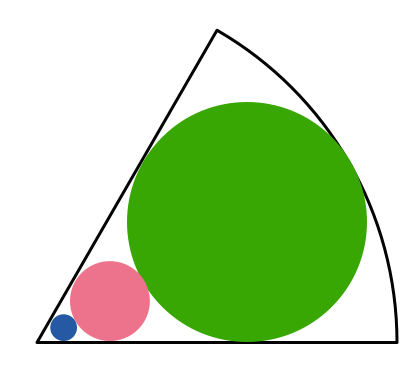# Circulisious

Geometry Level 3Given a sector of radius 10 and angle $\frac{ \pi}{3}$, we draw the largest circle (in green) that is tangent to all sides of the sector. We then draw the circle (in pink) that is tangent to the 2 sides, and also to the green circle. We repeat this process indefinately, where the next circle is shown in blue.

Find the sum of the areas of these infinitely many circles.

×